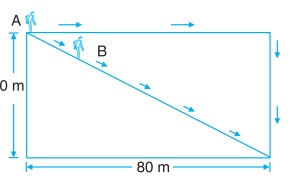# Velocity

When motion is along the shortest path, it is directed from the point of start to the point of finish. How fast this motion is determines the velocity. The velocity is the ratio of length of the shortest path (displacement) to the time taken.

Velocity = Displacement / Time taken

Velocity has same unit as the unit of speed - m s–1 (S.I. unit) or km h–1.

The shortest path or the displacement is directed from initial position of the object to the final position of the object. Hence, the velocity is also directed from initial position of the object to the final position of the object. Thus. the velocity has direction.

Speed does not have direction because it depends upon the total distance traveled by the object irrespective of the direction. The quantities which have direction are called vector and which do not have direction are called scalar quantity. Thus, velocity can also be expressed as

Velocity = Change in position / Time taken

Example: In a rectangular field of sides 60 m and 80 m respectively two farmers start moving from the same point and takes same time i.e. 30 minutes to reach diagonally opposite point along two different paths as shown in Figure. Find the velocity and speed of both the farmers.The displacement of both the farmer in same.

= √(602 + 802)

= 100 m

Velocity A and B = displacement / time taken = 100 / (30 × 60)

= 1/18 ms–1

Speed of A = Distance traveled / time taken = (80 + 60)/(30 × 60)

= 14/18 ms–1

Speed of B = Distance traveled / time taken = 100/(30 × 60) = 1/18 ms-1